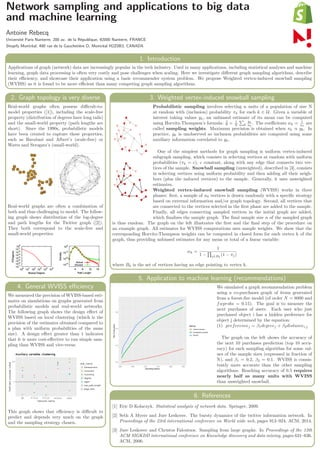Successfully reported this slideshow.

# Network sampling and applications to big data and machine learning

0

Share×
1 of 1
1 of 1

# Network sampling and applications to big data and machine learning

0

Share

Poster presented at the 2019 Montreal AI Symposium

Poster presented at the 2019 Montreal AI Symposium

## More Related Content

### Related Books

Free with a 14 day trial from Scribd

See all

### Related Audiobooks

Free with a 14 day trial from Scribd

See all

### Network sampling and applications to big data and machine learning

1. 1. Network sampling and applications to big data and machine learning Antoine Rebecq Université Paris Nanterre, 200 av. de la République, 92000 Nanterre, FRANCE Shopify Montréal, 490 rue de la Gauchetière O, Montréal H2Z0B3, CANADA 1. Introduction Applications of graph (network) data are increasingly popular in the tech industry. Used in many applications, including statistical analyses and machine learning, graph data processing is often very costly and pose challenges when scaling. Here we investigate diﬀerent graph sampling algorithms, describe their eﬃciency, and showcase their application using a basic recommender system problem. We propose Weighted vertex-induced snowball sampling (WVISS) as it is found to be more eﬃcient than many competing graph sampling algorithms. 2. Graph topology is very diverse Real-world graphs often possess diﬃcult-to- model properties (), including the scale-free property (distribution of degrees have long tails) and the small-world property (path lengths are short). Since the 1990s, probabilistic models have been created to capture these properties, such as Barabasi and Albert’s (scale-free) or Watts and Strogatz’s (small-world). Real-world graphs are often a combination of both and thus challenging to model. The follow- ing graph shows distribution of the log-degree and path lengths for the Twitter graph (). They both correspond to the scale-free and small-world properties: 4. General WVISS eﬃciency We measured the precision of WVISS-based esti- mates on simulations on graphs generated from probabilistic models and real-world networks. The following graph shows the design eﬀect of WVISS based on local clustering (which is the precision of the estimates obtained compared to a plan with uniform probabilities of the same size). A design eﬀect greater than 1 indicates that it is more cost-eﬀective to run simple sam- pling than WVISS and vice-versa: This graph shows that eﬃciency is diﬃcult to predict and depends very much on the graph and the sampling strategy chosen. 3. Weighted vertex-induced snowball sampling Probabilistic sampling involves selecting n units of a population of size N at random with (inclusion) probability πk for each k ∈ U. Given a variable of interest taking values yk, an unbiased estimate of its mean can be computed using Horvitz-Thompson’s formula: ˆ¯y = 1 N k yk πk . The coeﬃcients wk = 1 πk are called sampling weights. Maximum precision is obtained when πk ∝ yk. In practice, yk is unobserved so inclusion probabilities are computed using some auxiliary information correlated to yk. One of the simplest methods for graph sampling is uniform vertex-induced subgraph sampling, which consists in selecting vertices at random with uniform probabilities (πk = c), c constant, along with any edge that connects two ver- tices of the sample. Snowball sampling (unweighted), described in , consists in selecting vertices using uniform probability and then adding all their neigh- bors (plus the induced vertices) to the sample. Generally, it uses unweighted estimates. Weighted vertex-induced snowball sampling (WVISS) works in three phases: ﬁrst, a sample of n0 vertices is drawn randomly with a speciﬁc strategy based on external information and/or graph topology. Second, all vertices that are connected to the vertices selected in the ﬁrst phase are added to the sample. Finally, all edges connecting sampled vertices in the initial graph are added, which ﬁnalizes the sample graph. The ﬁnal sample size n of the sampled graph is thus random. The graph on the left illustrates the ﬁrst and the ﬁnal step of the procedure on an example graph. All estimates for WVISS computations uses sample weights. We show that the corresponding Horvitz-Thompson weights can be computed in closed form for each vertex k of the graph, thus providing unbiased estimates for any mean or total of a linear variable: wk = 1 1 − j∈Bk (1 − πj) where Bk is the set of vertices having an edge pointing to vertex k. 5. Application to machine learning (recommendations) 0.0 0.2 0.4 0.6 0.00 0.05 0.10 0.15 0.20 0.25 Sampling fraction Top10accuracy Method Uniform induced Unweighted snowball WVISS We simulated a graph recommendation problem using a co-purchases graph of items generated from a forest-ﬁre model (of order N = 8000 and fwprobs = 0.15). The goal is to measure the next purchases of users. Each user who just purchased object i has a hidden preference for object j determined by the equation: (1) preferencej = β1degreej + β2distancei,j The graph on the left shows the accuracy of the next 10 purchases prediction (top 10 accu- racy) for each sampling algorithm for some val- ues of the sample sizes (expressed in fraction of N), and β1 = 0.2, β2 = 0.1. WVISS is consis- tently more accurate than the other sampling algorithms. Reaching accuracy of 0.5 requires nearly half as many units with WVISS than unweighted snowball. 6. References  Eric D Kolaczyk. Statistical analysis of network data. Springer, 2009.  Seth A Myers and Jure Leskovec. The bursty dynamics of the twitter information network. In Proceedings of the 23rd international conference on World wide web, pages 913–924. ACM, 2014.  Jure Leskovec and Christos Faloutsos. Sampling from large graphs. In Proceedings of the 12th ACM SIGKDD international conference on Knowledge discovery and data mining, pages 631–636. ACM, 2006.